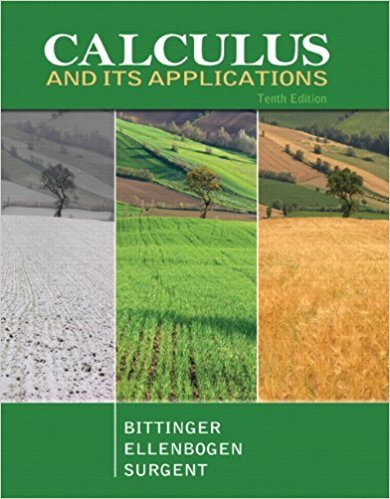×
×

# Solutions for Chapter R.3: Finding Domain and Range## Full solutions for Calculus and Its Applications | 10th Edition

ISBN: 9780321694331Solutions for Chapter R.3: Finding Domain and Range

Solutions for Chapter R.3
4 5 0 252 Reviews
21
3
##### ISBN: 9780321694331

Calculus and Its Applications was written by and is associated to the ISBN: 9780321694331. This textbook survival guide was created for the textbook: Calculus and Its Applications, edition: 10. Since 67 problems in chapter R.3: Finding Domain and Range have been answered, more than 24060 students have viewed full step-by-step solutions from this chapter. This expansive textbook survival guide covers the following chapters and their solutions. Chapter R.3: Finding Domain and Range includes 67 full step-by-step solutions.

Key Calculus Terms and definitions covered in this textbook

If u < v , then u + w < v + w

• Arccotangent function

See Inverse cotangent function.

• Common difference

See Arithmetic sequence.

• Coordinate plane

See Cartesian coordinate system.

• Direct variation

See Power function.

• Division algorithm for polynomials

Given ƒ(x), d(x) ? 0 there are unique polynomials q1x (quotient) and r1x(remainder) ƒ1x2 = d1x2q1x2 + r1x2 with with either r1x2 = 0 or degree of r(x) 6 degree of d1x2

• Explanatory variable

A variable that affects a response variable.

• Hypotenuse

Side opposite the right angle in a right triangle.

• Irrational numbers

Real numbers that are not rational, p. 2.

• Leaf

The final digit of a number in a stemplot.

• Linear system

A system of linear equations

• Measure of an angle

The number of degrees or radians in an angle

• Obtuse triangle

A triangle in which one angle is greater than 90°.

• Parametric equations

Equations of the form x = ƒ(t) and y = g(t) for all t in an interval I. The variable t is the parameter and I is the parameter interval.

• Partial sums

See Sequence of partial sums.

• Polar coordinates

The numbers (r, ?) that determine a point’s location in a polar coordinate system. The number r is the directed distance and ? is the directed angle

• Positive numbers

Real numbers shown to the right of the origin on a number line.

• Secant line of ƒ

A line joining two points of the graph of ƒ.

• Transverse axis

The line segment whose endpoints are the vertices of a hyperbola.

• Vertices of a hyperbola

The points where a hyperbola intersects the line containing its foci.

×